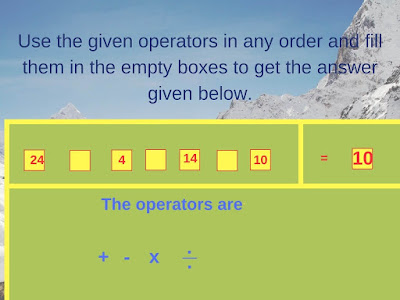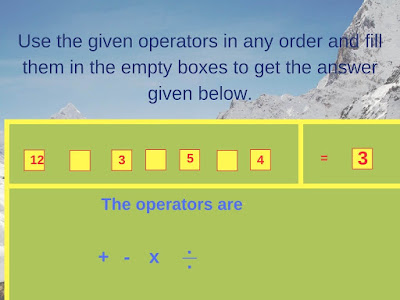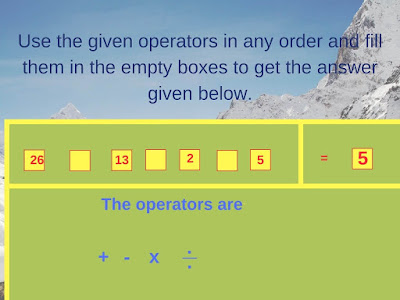## Sunday, December 15, 2019

Solving the mathematical equations is very interesting brain teasers. If the fun factor is added in these math equations then one will love to solve these problems. This fun factor can be added by replacing numbers with the pictures or removing the operators. Here we are presenting few math equations in which the operator has been removed.
In each of these math problems, operators in the equations are removed. The list of the operators which can be used to solve the equation is given in each of the problems. Each operator can be used as many times as one wish. However, BODMAS rules apply in each of the equations.1. Can you put operators and make this maths equation correct?2. Make this equation correct by putting the operators.3. Can you make this equation true after adding given operators?4. Can you correct this Maths Equations by adding operators in blank spaces?5. Fill in the Blanks with given operators to make this equation correct.

Solve the following listed Fun Brain Teasers and Puzzles
List of Fun Brain Teasers and Puzzles

1. Fun Puzzles with answers that will blow your mind: Do checkout these Fun Puzzles which will twist and give the challenge to your mind.

2. Brain Maths Logic Puzzles for Teens: Use your Mathematical and Logical skills to solve these fun puzzles.

3. Brain Buster Math Questions and Answers: These are fun Maths Puzzles in which some numbers are given around a triangle. These numbers are related to reaching other logically and mathematically. Your challenge is to find these relations and solve for the missing number.

4. Fun Brain Teasers For Kids, Teens and Adults with Answers: These are Fun Brain Teasers which are a mix of Mathematical, Logical and Word Brain Teasers.

1.1.